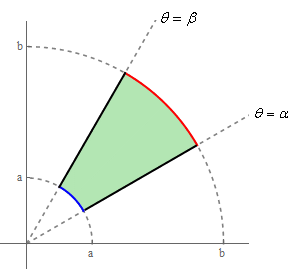Paul's Online Notes
Home / Calculus III / Multiple Integrals / Double Integrals in Polar Coordinates
Show Mobile Notice Show All Notes Hide All Notes
Mobile Notice
You appear to be on a device with a "narrow" screen width (i.e. you are probably on a mobile phone). Due to the nature of the mathematics on this site it is best views in landscape mode. If your device is not in landscape mode many of the equations will run off the side of your device (should be able to scroll to see them) and some of the menu items will be cut off due to the narrow screen width.
Assignment Problems Notice
Please do not email me to get solutions and/or answers to these problems. I will not give them out under any circumstances nor will I respond to any requests to do so. The intent of these problems is for instructors to use them for assignments and having solutions/answers easily available defeats that purpose.

### Section 15.4 : Double Integrals in Polar Coordinates

1. Evaluate $$\displaystyle \iint\limits_{D}{{3x{y^2} - 2\,dA}}$$ where $$D$$ is the unit circle centered at the origin.
2. Evaluate $$\displaystyle \iint\limits_{D}{{4x - 2y\,dA}}$$ where $$D$$ is the top half of region between $${x^2} + {y^2} = 4$$ and $${x^2} + {y^2} = 25$$.
3. Evaluate $$\displaystyle \iint\limits_{D}{{6xy + 4{x^2}\,dA}}$$ where $$D$$ is the portion of $${x^2} + {y^2} = 9$$ in the 2nd quadrant.
4. Evaluate $$\displaystyle \iint\limits_{D}{{\sin \left( {3{x^2} + 3{y^2}} \right)\,dA}}$$ where $$D$$ is the region between $${x^2} + {y^2} = 1$$ and $${x^2} + {y^2} = 7$$.
5. Evaluate $$\displaystyle \iint\limits_{D}{{{{\bf{e}}^{1 - {x^{\,2}} - {y^{\,2}}}}\,dA}}$$ where $$D$$ is the region in the 4th quadrant between $${x^2} + {y^2} = 16$$ and $${x^2} + {y^2} = 36$$.
6. Use a double integral to determine the area of the region that is inside $$r = 6 - 4\cos \theta$$.
7. Use a double integral to determine the area of the region that is inside $$r = 4$$ and outside $$r = 8 + 6\sin \theta$$.
8. Evaluate the following integral by first converting to an integral in polar coordinates. $\int_{{ - 2}}^{0}{{\int_{{ - \sqrt {4 - {y^{\,2}}} }}^{{\sqrt {4 - {y^{\,2}}} }}{{\,\,\,{x^2}\,dx}}\,dy}}$
9. Evaluate the following integral by first converting to an integral in polar coordinates. $\int_{{ - 1}}^{1}{{\int_{0}^{{\sqrt {1 - {x^{\,2}}} }}{{\,\,\,\sqrt {{x^2} + {y^2}} \,dy}}\,dx}}$
10. Use a double integral to determine the volume of the solid that is below $$z = 9 - 4{x^2} - 4{y^2}$$ and above the $$xy$$-plane.
11. Use a double integral to determine the volume of the solid that is bounded by $$z = 12 - 3{x^2} - 3{y^2}$$ and $$z = {x^2} + {y^2} - 8$$.
12. Use a double integral to determine the volume of the solid that is inside both the cylinder $${x^2} + {y^2} = 9$$ and the sphere $${x^2} + {y^2} + {z^2} = 16$$.
13. Use a double integral to derive the area of a circle of radius $$a$$.
14. Use a double integral to derive the area of the region between circles of radius a and b with $$\alpha \le \theta \le \beta$$. See the image below for a sketch of the region.